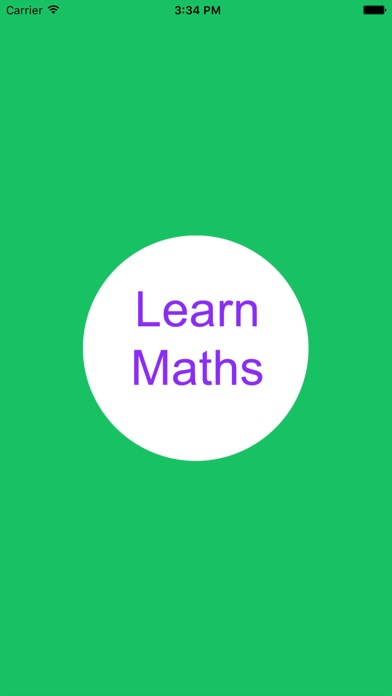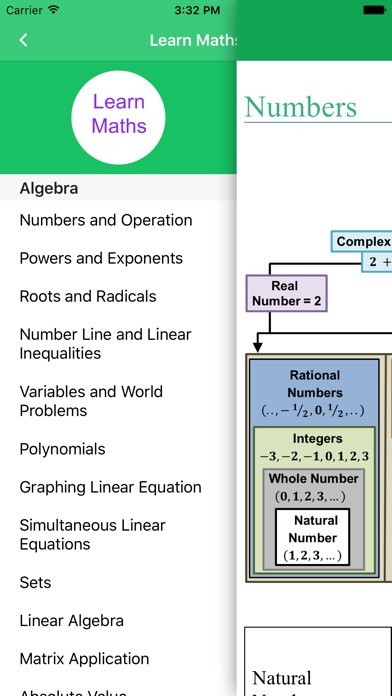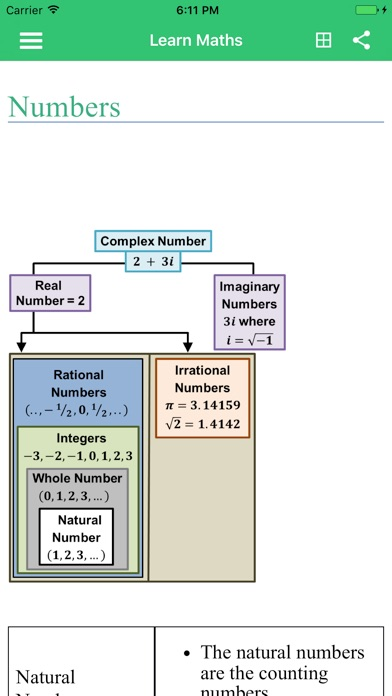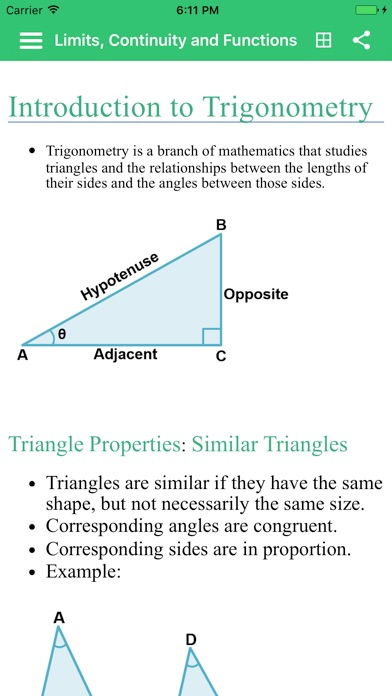This app was removed from the App Store.Learn Maths Full

iOS UniversalEducation

Free

This application help you learn or revise your knowledge about Algebra, Calculus, Trigonometry, Statistics!!

• Numbers and Operation
• Powers and Exponents
• Roots and Radicals
• Number Line and Linear Inequalities
• Variables and Word Problems
• Polynomials
• Graphing Linear Equation
• Simultaneous Linear Equations
• Sets
• Linear Algebra
• Matrix Application
• Absolute Value
• And more contents about Calculus, Trigonometry, Statistics...

What's New

- Minor bug fixes

•••••